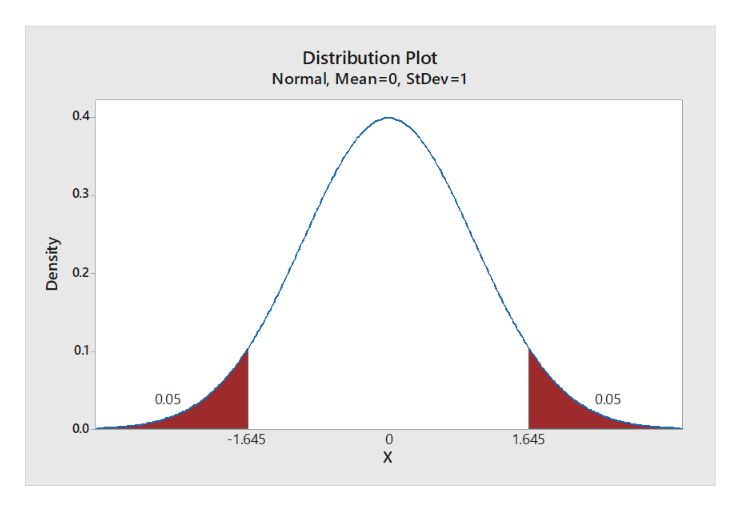# You want to obtain a sample to estimate a population mean. Based on previous evidence, you believe the population standard deviation is approximately σ=37.8σ=37.8. You would like to be 90% confident that your estimate is within 1 of the true population mean. How large of a sample size is required?

Question
2 views

You want to obtain a sample to estimate a population mean. Based on previous evidence, you believe the population standard deviation is approximately σ=37.8σ=37.8. You would like to be 90% confident that your estimate is within 1 of the true population mean. How large of a sample size is required?

check_circle

Step 1

Step-by-step procedure to obtain the value of z using MINITAB:

• Choose Graph > Probability Distribution Plot choose View Probability > OK.
• From Distribution, choose ‘Normal’ distribution.
• Choose Probability and Both Tail for the region of the curve to shade.
• Enter the Probability value as 10.
• Click OK.

Output using the MINITAB software is given below:help_outlineImage TranscriptioncloseDistribution Plot Normal, Mean=0, StDev=1 0.4 0.3 0.2 0.1 0.05 0.05 0.0 -1.645 1.645 X Density fullscreen
Step 2

From the output, the value of z is 1.645 for 90% confidence...

### Want to see the full answer?

See Solution

#### Want to see this answer and more?

Solutions are written by subject experts who are available 24/7. Questions are typically answered within 1 hour.*

See Solution
*Response times may vary by subject and question.
Tagged in

### Hypothesis Testing